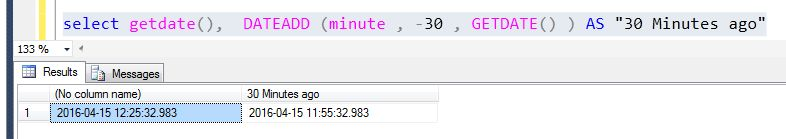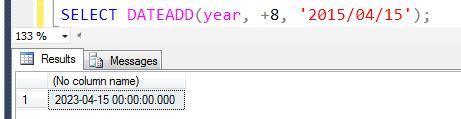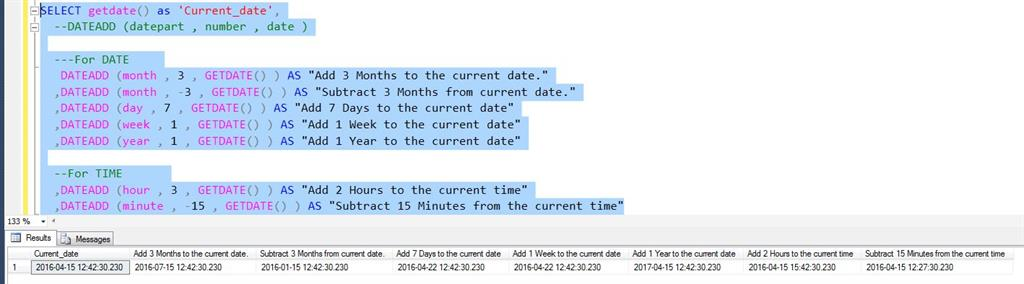In focus

# How To Use DATEADD Function

## In this article we will learn how to use DATEADD system function. Sometimes we need to get new datetime values from existing date records.

Gagan Sharma Apr 20, 2016

Sometimes we need to get new datetime values from existing date records. In this case we need to use DATEADD function. This function requires three parameters.

Syntax:
The number variable adds specified datepart value to date variable. Number interval(integer) is signed integer. It can be negative.

Query 1:  If we need to get 30 minute ago. We must use this following query.
1. select getdate(), DATEADD (minute , -30 , GETDATE() ) AS "30 Minutes ago"
Output:  The getdate() function will display the current time and date but DATEADD is use to get the desired output.Query 2: In place of using getdate function we can manually provide date as input.

Output:Query 3:
1. SELECT getdate() as 'Current_date',
2.   --DATEADD (datepart , number , date )
3.
4.   ---For DATE
5.    DATEADD (month , 3 , GETDATE() ) AS "Add 3 Months to the current date."
6.   ,DATEADD (month , -3 , GETDATE() ) AS "Subtract 3 Months from current date."
7.   ,DATEADD (day , 7 , GETDATE() ) AS "Add 7 Days to the current date"
8.   ,DATEADD (week , 1 , GETDATE() ) AS "Add 1 Week to the current date"
9.   ,DATEADD (year , 1 , GETDATE() ) AS "Add 1 Year to the current date"
10.
11.   --For TIME
12.   ,DATEADD (hour , 3 , GETDATE() ) AS "Add 2 Hours to the current time"
13.   ,DATEADD (minute , -15 , GETDATE() ) AS "Subtract 15 Minutes from the current time"
Output: